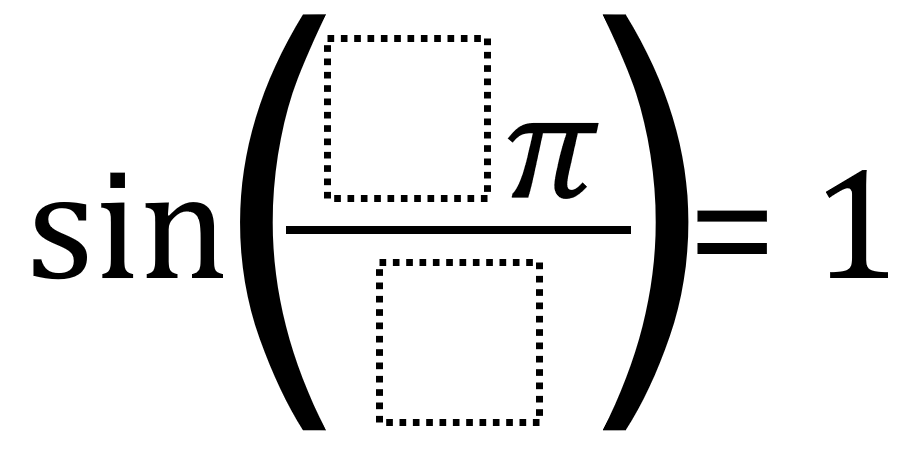# Evaluating Trigonometric Functions 1

Directions: Using the digits 1 to 9 at most one time each, place a digit in each box to make five true number sentences. You may reuse all the digits for each number sentence.### Hint

If you could use any digits you wanted (and not just the digits 1 to 9), how many possible true number sentences could you make? How can you determine if a number sentence is true?